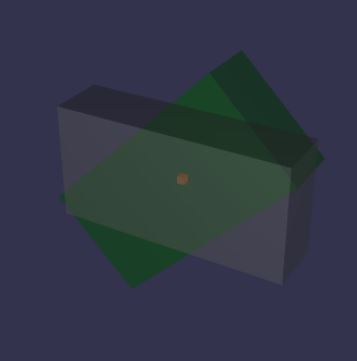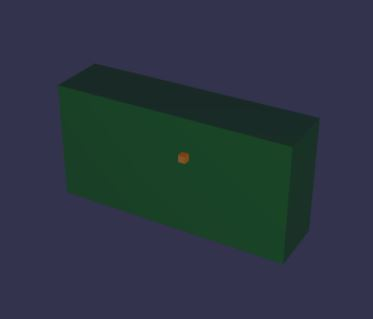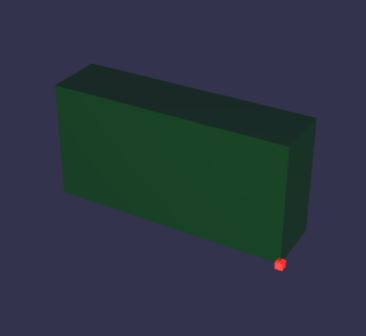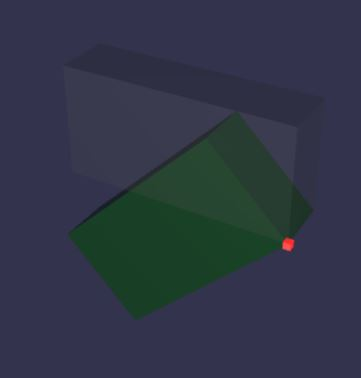# Using a Pivot

In all the diagrams the red box gives the local origin, the grey box shows the intial created position of the box to tranform and the green box the transformed box.

Rotations of a mesh always take place around its local origin. In other words the local origin of the mesh is the pivot of the mesh.There are times when you would like to rotate a mesh around a different pivot point.

This is achieved NOT by translating the local origin onto the pivot point BUT by translating the mesh in the opposite direction so that the local origin is not re-positioned within the world axes.

## Setting the Pivot Matrix.

A pivot is set using a matrix that when applied to the mesh translates it relative to the local origin.

The method needed is

``````mesh.setPivotMatrix(BABYLON.Matrix.Translation(x, y, z));
``````

### Example

Create a box.You now want the box to rotate not about the centre but about the front lower right corner, which has coordinates (4, -2, -1).

To place the pivot point as required a translation of (-4, 2, 1) is needed. This is done using

``````greenBox.setPivotMatrix(BABYLON.Matrix.Translation(-4, 2, 1));
``````

GivingIf you now want the box back in its starting position you will need to place the local origin of the box at (4, -2, -1) to take into account the translation of the box relative to the pivot.Now when you apply a rotation it will be about the pivot## Aligning Pivoted Meshes

Ordinarily it is easy enough to align the centres of meshes because the position of each of their local origins can be set relative to the world origin.
However when a pivot has been applied to the meshes each mesh will already have been translated and their local origins are no longer at their centres.

If the centre of a pivoted mesh is to be positioned at (xc, yc, zc) and the pivot was positioned using the translation (-xt, -yt, -zt) then the centre of the mesh can be placed using

``````var centreAt = new BABYLON.Vector3(xc, yc, zc);
var pivotAt = new BABYLON.Vector3(xt, yt, zt);
``````

Playground Example - Aligned Pivoted Meshes Rotated -

## Setting Mesh and Pivot Position at the Same Time

When you want to position a mesh with its centre at (xc, yc, zc) and a pivot at (xp, yp, zp) just follow these steps

1. Position the mesh at the pivot cordinates;
2. Work out the translation that takes the pivot to the meshes centre (xc - xp, yc - yp, zc - zp);
3. Set the pivot matrix using this translation

In Javascript

``````var centreAt = new BABYLON.Vector3(xc, yc, zc);
var pivotAt = new BABYLON.Vector3(xp, yp, zp);
mesh.position = pivotAt;
var pivotTranslate = centreAt.substract(pivotAt);
mesh.setPivotMatrix(BABYLON.Matrix.Translation(pivotTranslate.x, pivotTranslate.y, pivotTranslate.z));
``````

The mesh will now be positioned with its centre at (xc, yc, zc) and all rotations and scaling will take place with reference to the pivot at (xp, yp, zp).

Playground Example - Scaling from a Pivot -

In the above playgrounds the sphere(s) allow you to vary the rotation and scaling and check that they do take place with reference to the pivot.

## Resetting the Pivot without Moving the Mesh.

When you want the mesh to stay in the same place but move the pivot to a new position (xp, yp, zp) you need to have stored and kept the current centre of the mesh. Then the steps are as above.

In Javascript

``````pivotAt = new BABYLON.Vector3(xp, yp, zp);
mesh.position = pivotAt;
pivotTranslate = centreAt.substract(pivotAt);  //centreAt retained from previous pivot setting
mesh.setPivotMatrix(BABYLON.Matrix.Translation(pivotTranslate.x, pivotTranslate.y, pivotTranslate.z));
``````

The following sequence of playgrounds goes from setting the first pivot position to scaling about the second pivot position

Playground Example - Set Second Pivot -

Playground Example - Scaling from Second Pivot -

NOTE: Restting the pivot this way resets it relative to the unrotated mesh. That is if the mesh has been rotated before resetting the pivot the actual order remains reset pivot do rotation.

The following sequence of playgrounds shows setting the first pivot, rotating the pivot then resetting the pivot.

Playground Example - Rotate About First Pivot -

Playground Example - Set Second Pivot -

## Set and Get a Pivot Point

There are three useful functions to aid setting and getting a pivot point. These are

``````mesh.setPivotPoint(Vector3);
mesh.getPivotPoint(); // returns Vector3
mesh.getAbsolutePivotPoint(); // returns Vector3
``````

The following sequence of playgrounds goes from setting the first pivot point to scaling about the second pivot point

Playground Example - Set Second Pivot Point -

Playground Example - Scaling from Second Pivot Point -

It is possible to reset the pivot point and maintain the position and rotation of the mesh.

To do this the current rotation of the mesh has to be stored and its rotation set to (0, 0, 0) before the pivot point is reset. The current rotation is then re-applied to the mesh.

The following sequence of playgrounds shows setting the first pivot point, rotating the pivot then resetting the pivot point and re-applying the rotation.

Playground Example - Rotate About First Pivot Point -

Playground Example - Set Second Pivot Point and Rotate -